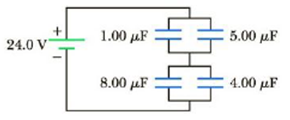Chapter 16, Problem 43P

Chapter
Section
Textbook Problem

Find the charge on each of the capacitors in Figure P16.43.Figure P16.43

To determine
The charge on each capacitor.

Explanation

The capacitors 1.00μF and 5.00μF are connected in series combination. The equivalent capacitance is,

Cpt=C1.00μFC5.00μFC1.00μF+C5.00μF

The capacitors 8.00μF and 4.00μF are connected in series combination. The equivalent capacitance is,

Cpb=C8.00μFC4.00μFC8.00μF+C4.00μF

The capacitances Cpt and Cpb are in parallel combination. The total equivalent capacitance is,

Ceq=Cpt+Cpb

Therefore,

Ceq=(C1.00μFC5.00μFC1.00μF+C5.00μF)+(C8.00μFC4.00μFC8.00μF+C4.00μF)

Substitute 1.00μF for C1.00μF , 5.00μF for C5.00μF , 8.00μF for C8.00μF and 4.00μF for C4.00μF

Ceq=[(1.00μF)(5.00μF)(1.00μF)+(5.00μF)]+[(8.00μF)(4.00μF)(8.00μF)+(4.00μF)]=4.00μF

Formula to calculate the total charge is,

Qtotal=CeqV

Substitute 4.00μF for Ceq and 24.0 V for V.

Qtotal=(4.00μF)(24.0V)=96.0μC

Formula to calculate the charge on 1.00μF capacitor is,

Q1=C1.00μF(QtotalC1.00μF+C5.00μF)

Substitute 96.0μC for Qtotal , 1.00μF for C1.00μF and 5.00μF for C5.00μF

Q1=(1

Still sussing out bartleby?

Check out a sample textbook solution.

See a sample solution

The Solution to Your Study Problems

Bartleby provides explanations to thousands of textbook problems written by our experts, many with advanced degrees!

Get Started

Find more solutions based on key concepts